# Frank Solutions for Class 10 Maths Chapter 1 Compound Interest

Frank Solutions for Class 10 Maths Chapter 1 Compound Interest are available here. These exercises are formulated by our subject experts in order to assist you with your exam preparation to attain good marks in Maths exams. Students who wish to score good marks in Maths should practise Frank Solutions for Class 10 Maths Chapter 1. This book is one of the top materials when it comes to providing a question bank to practise.

Chapter 1 – Compound Interest explains that it is the interest on a loan, which is calculated based on both the initial principal and the accumulated interest from previous periods. In the Frank Solutions for Class 10 Maths Chapter 1, students learn how to calculate interest.

## Download the PDF of Frank Solutions for Class 10 Maths Chapter 1 Compound Interest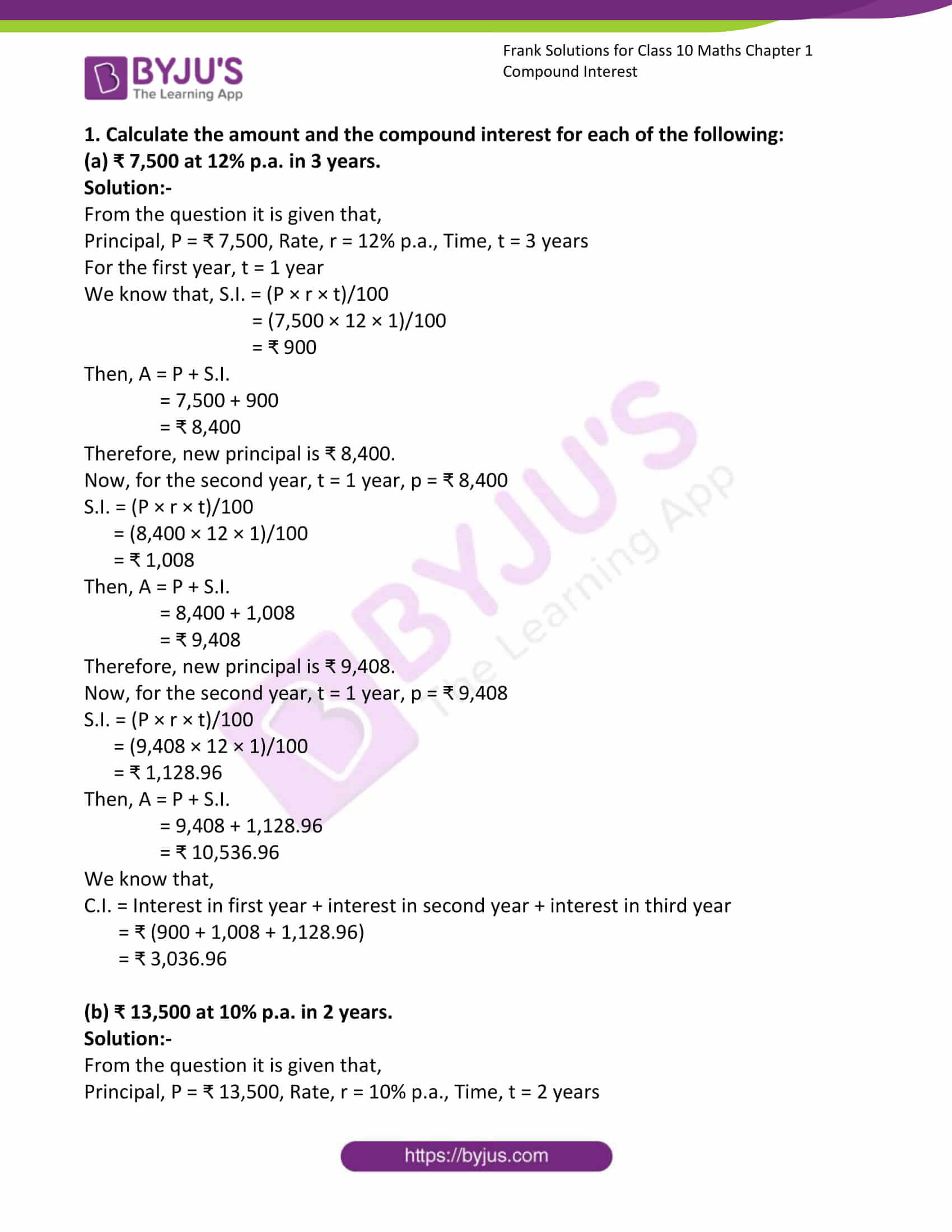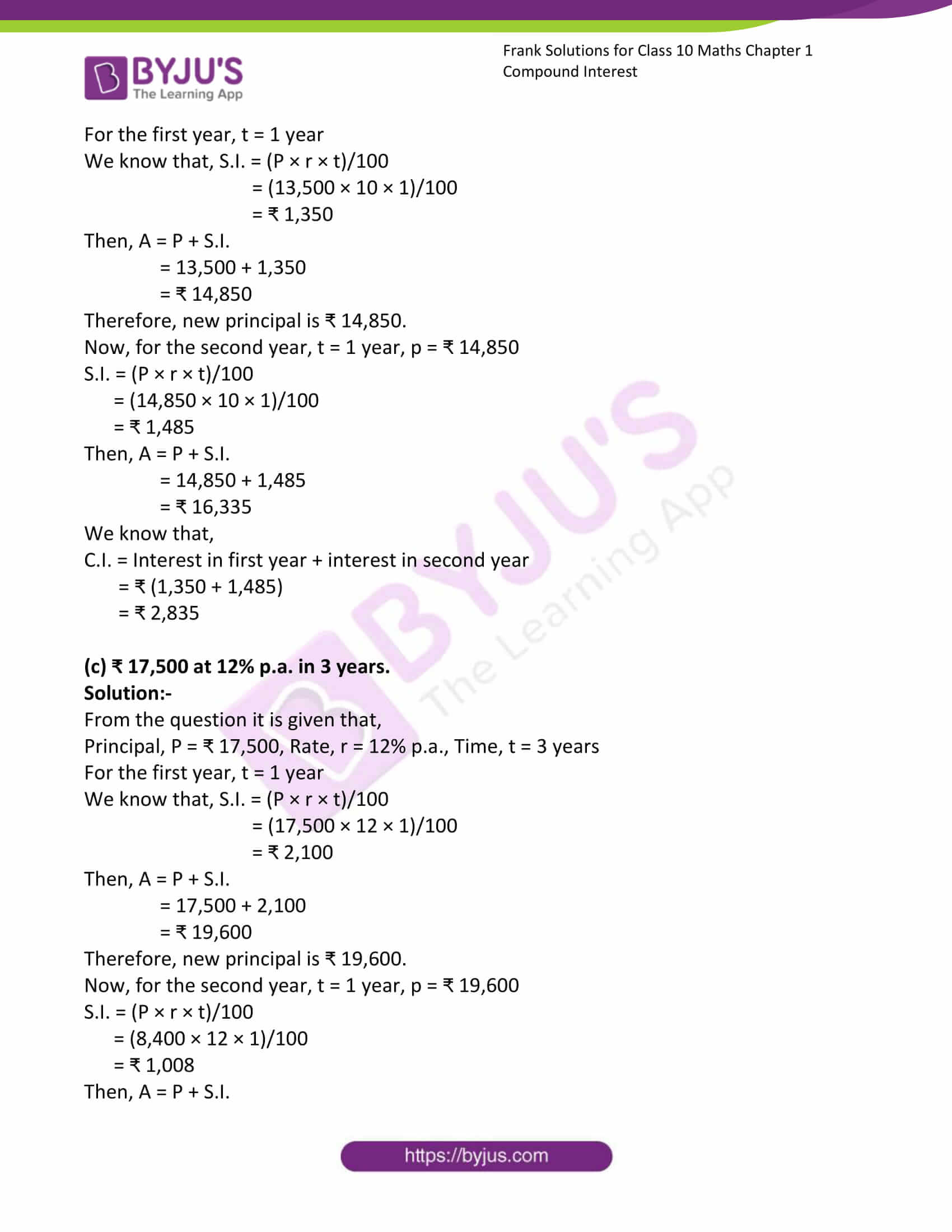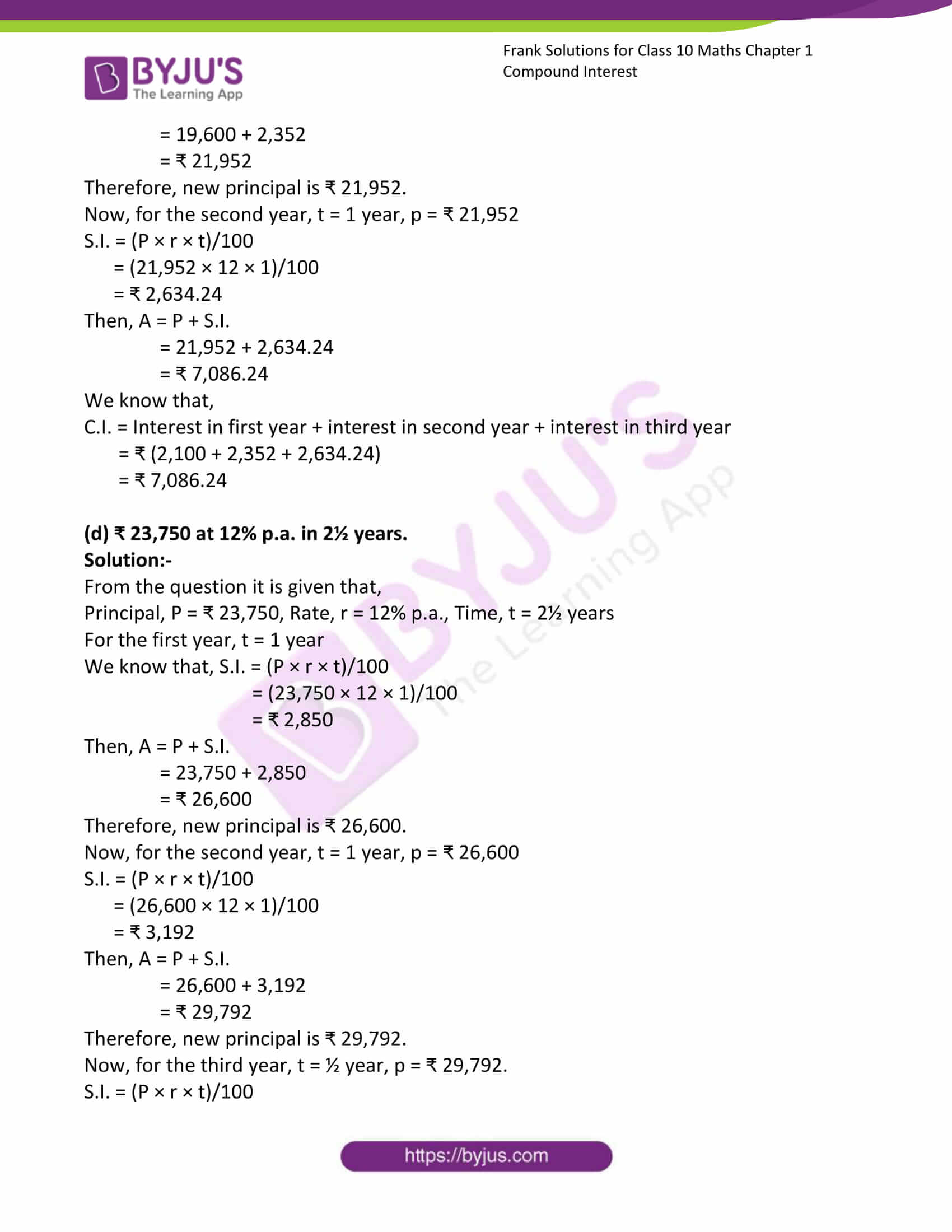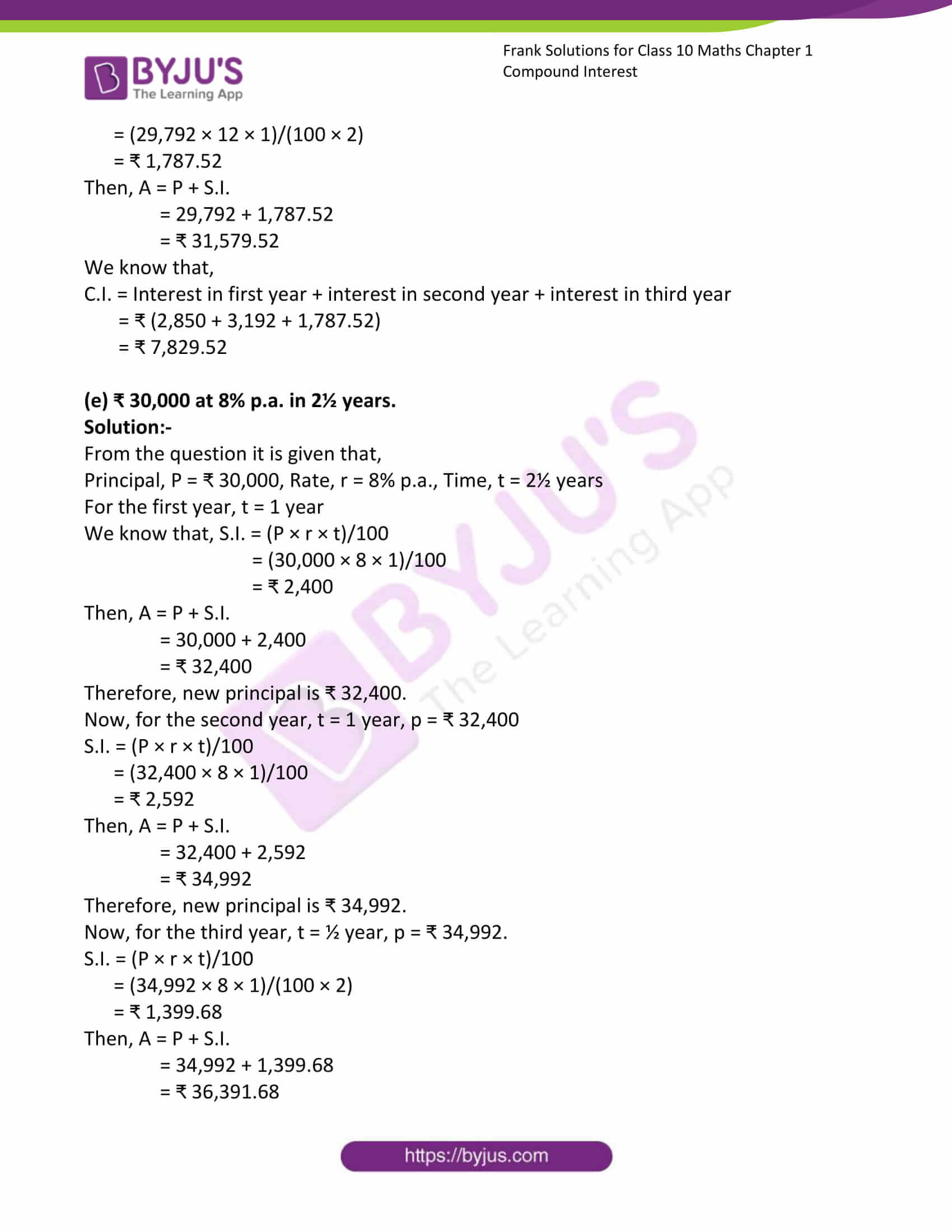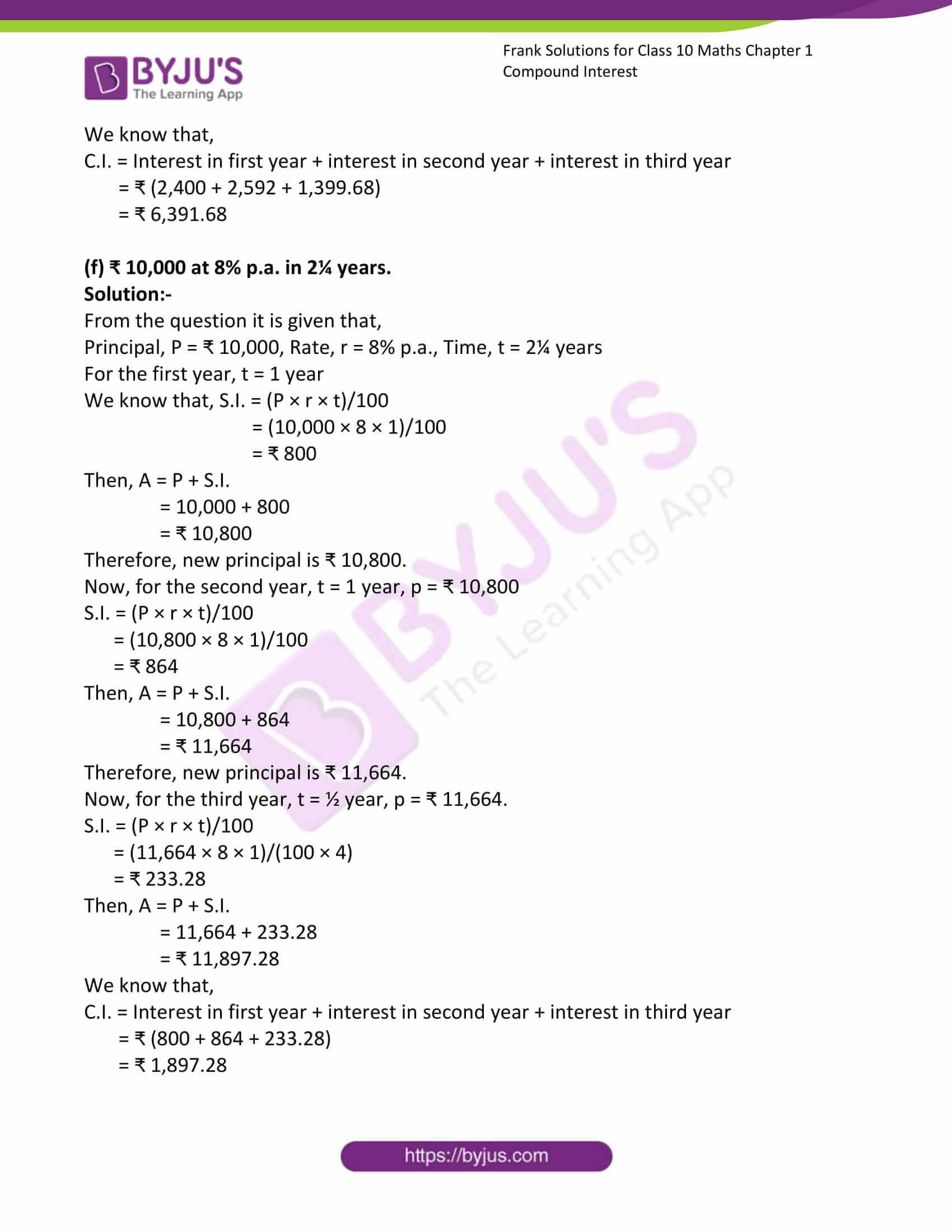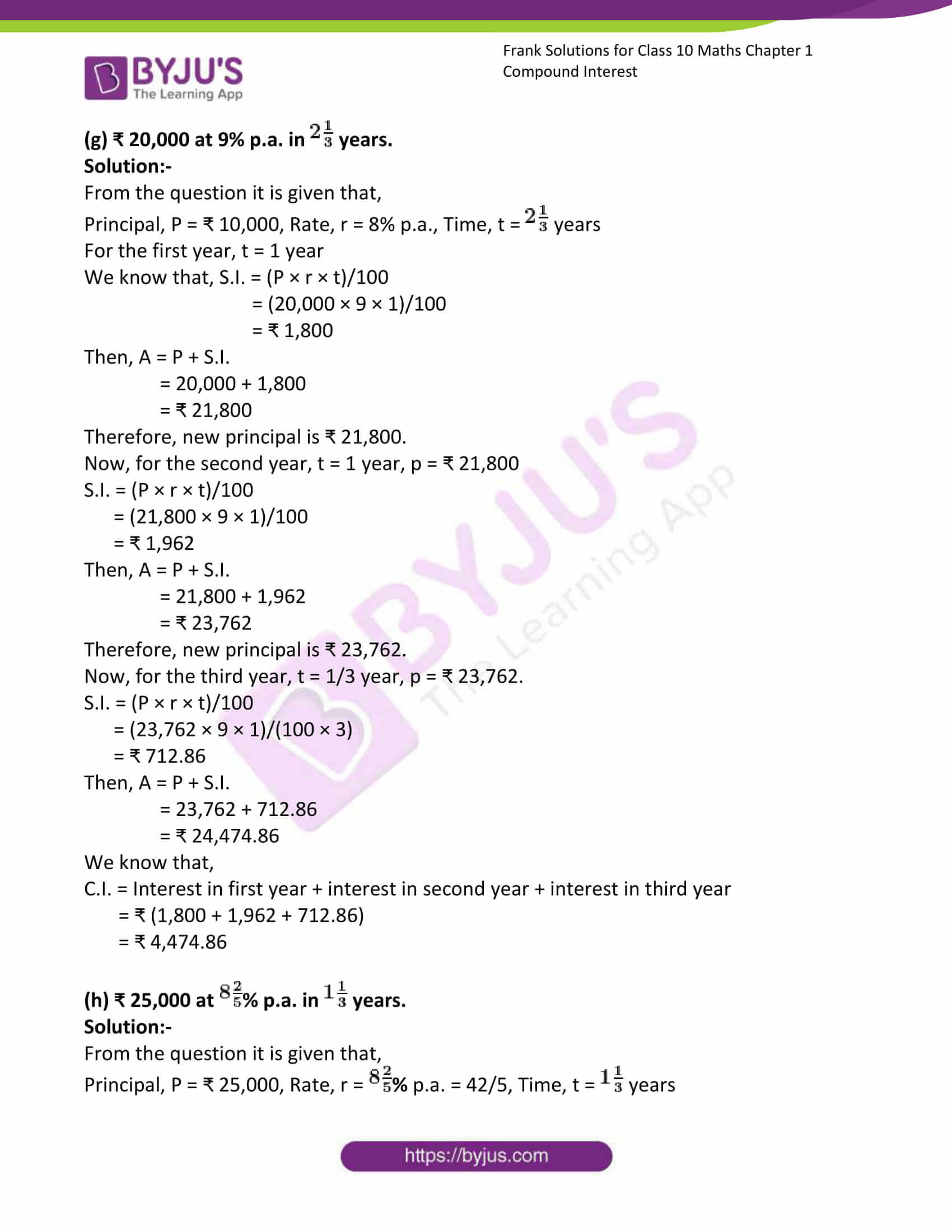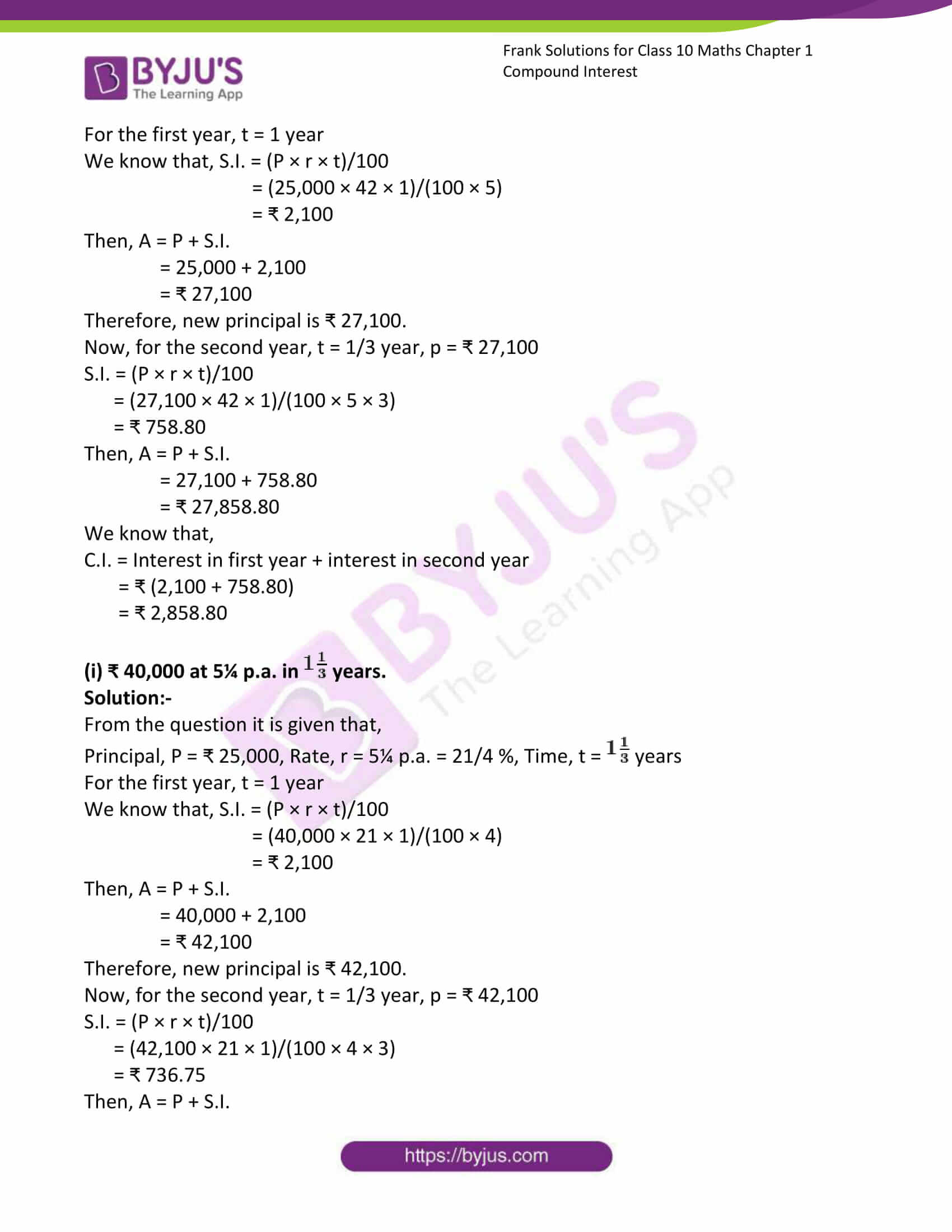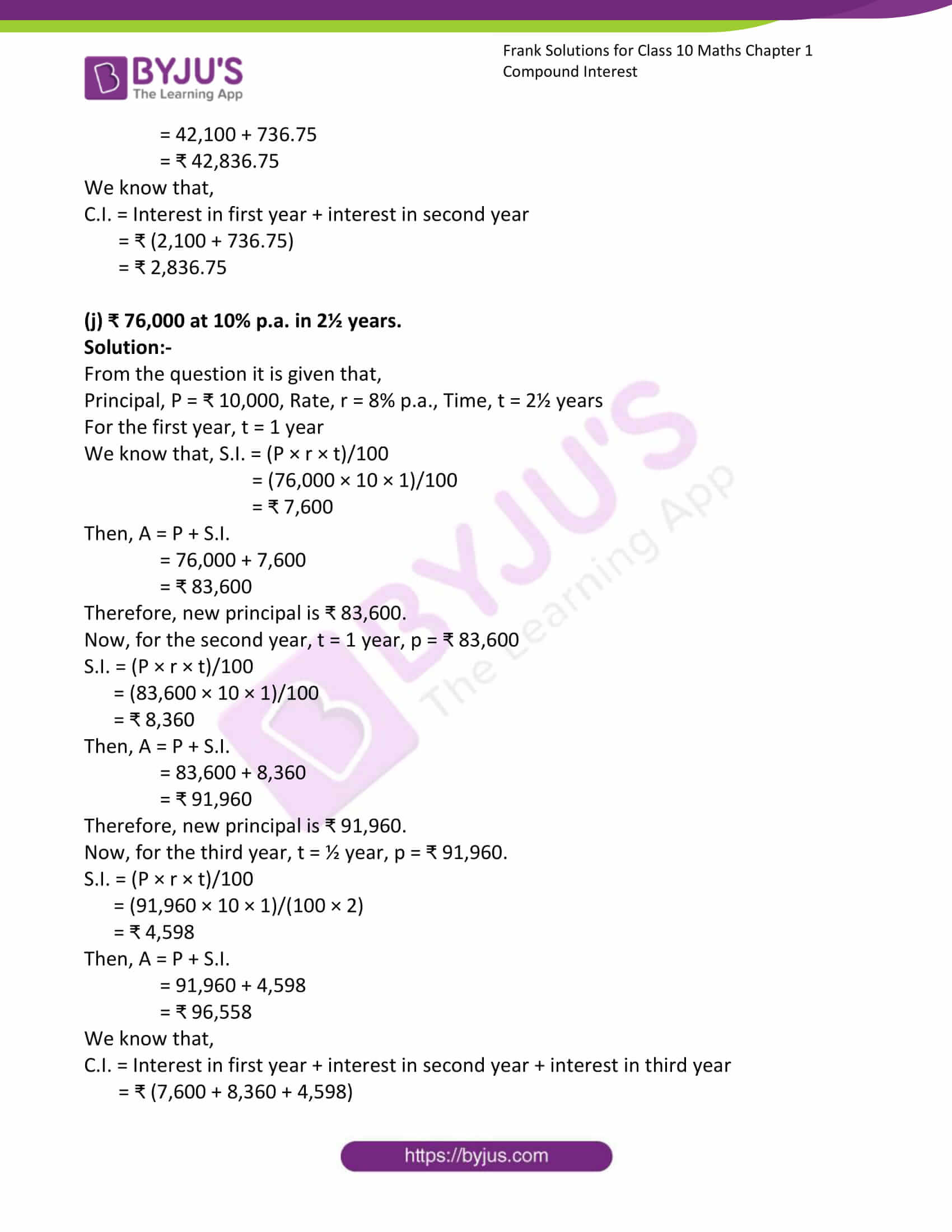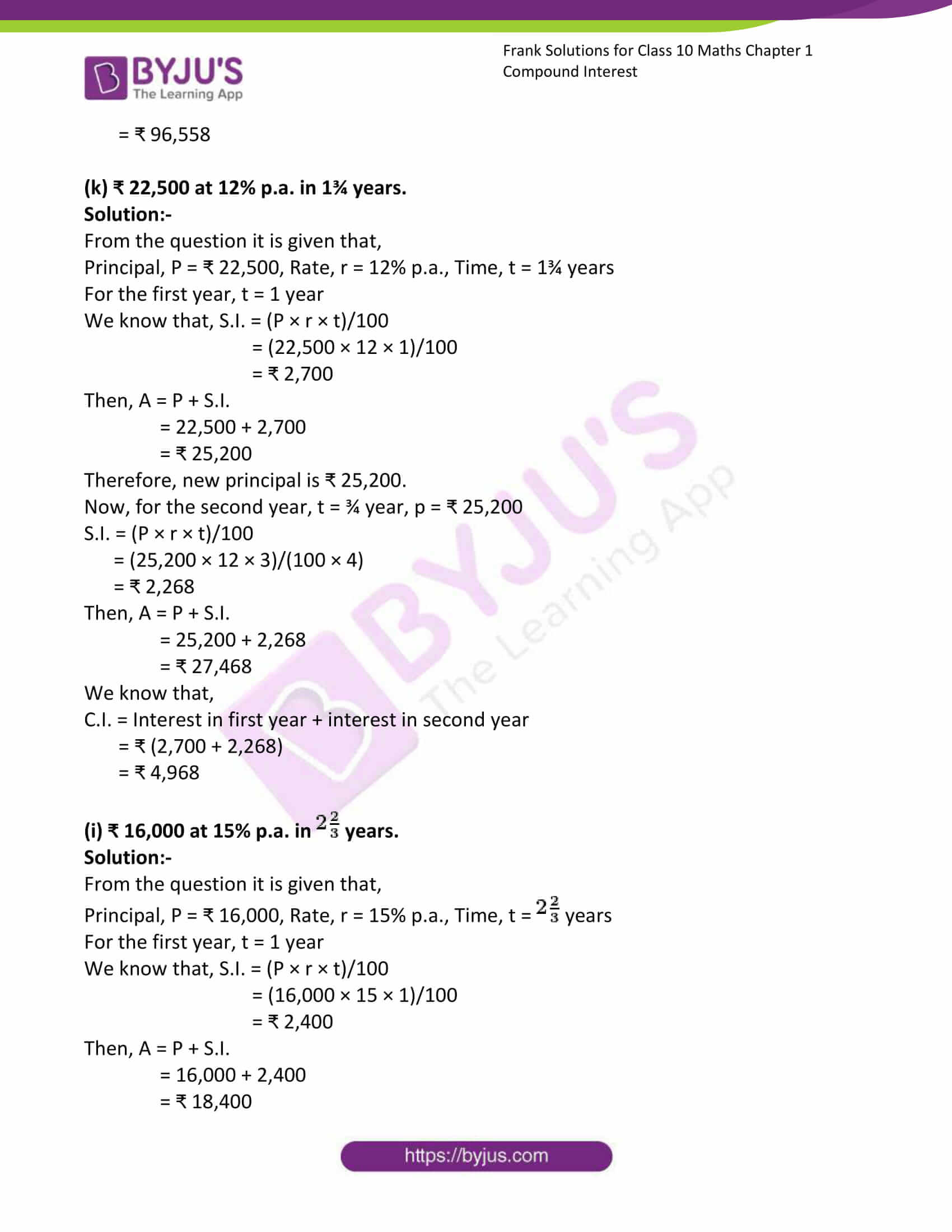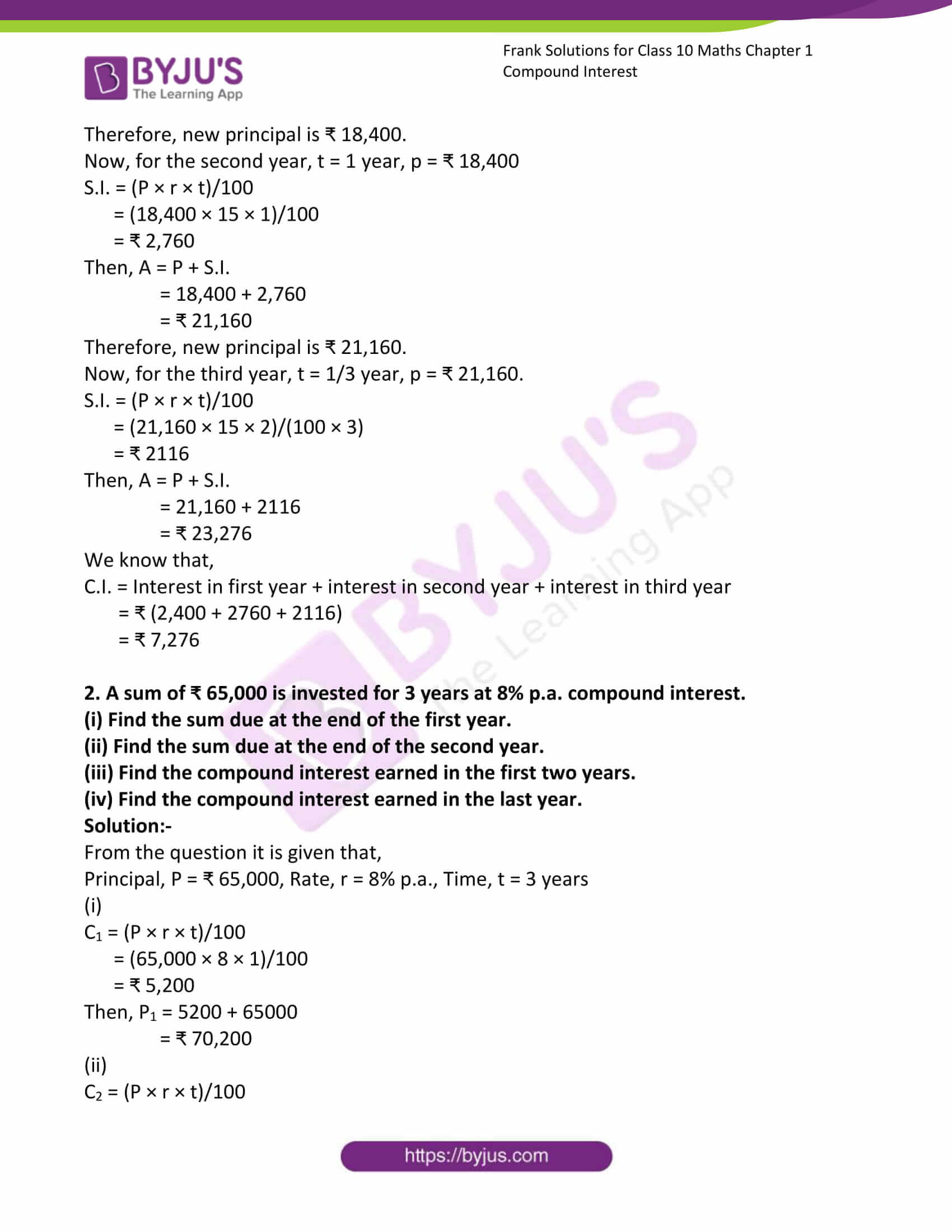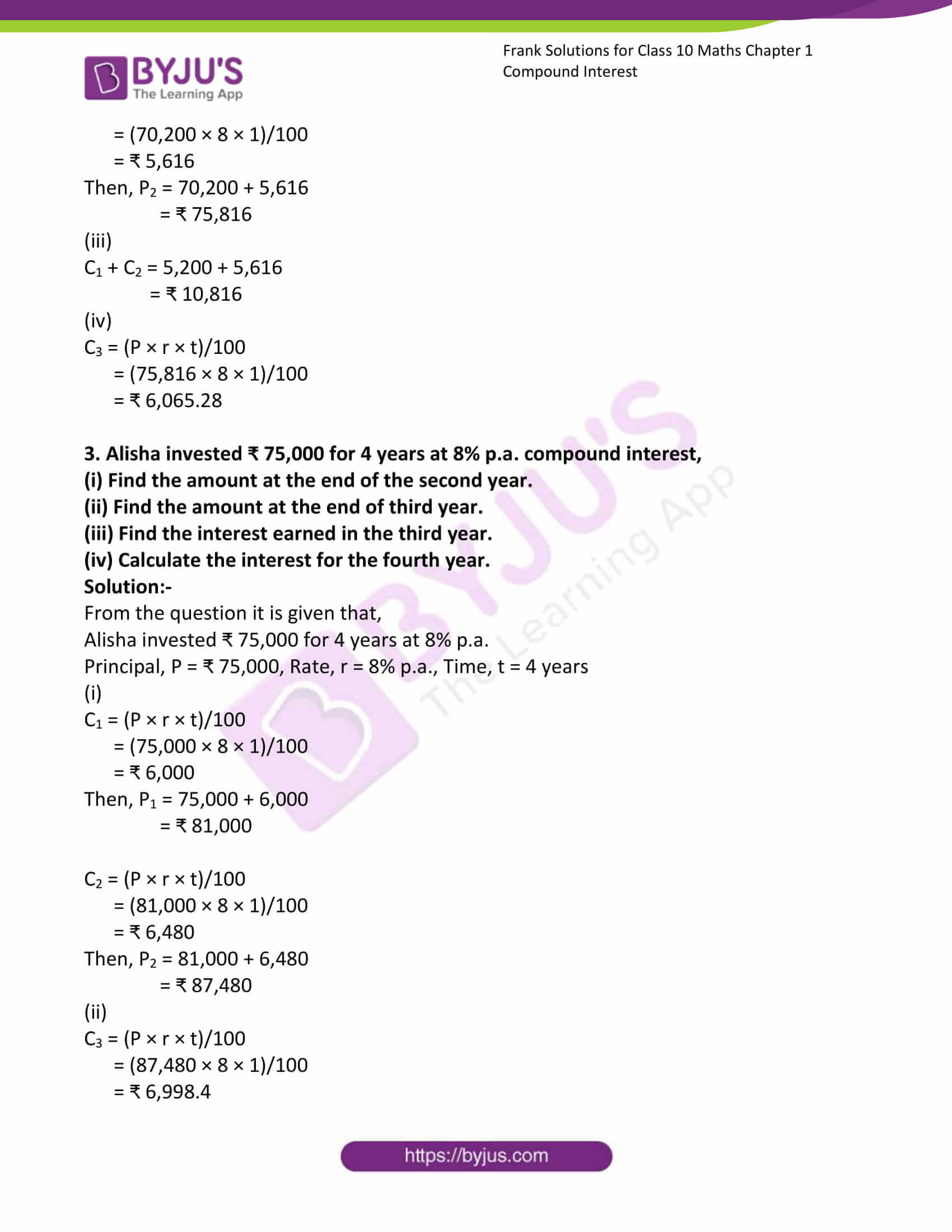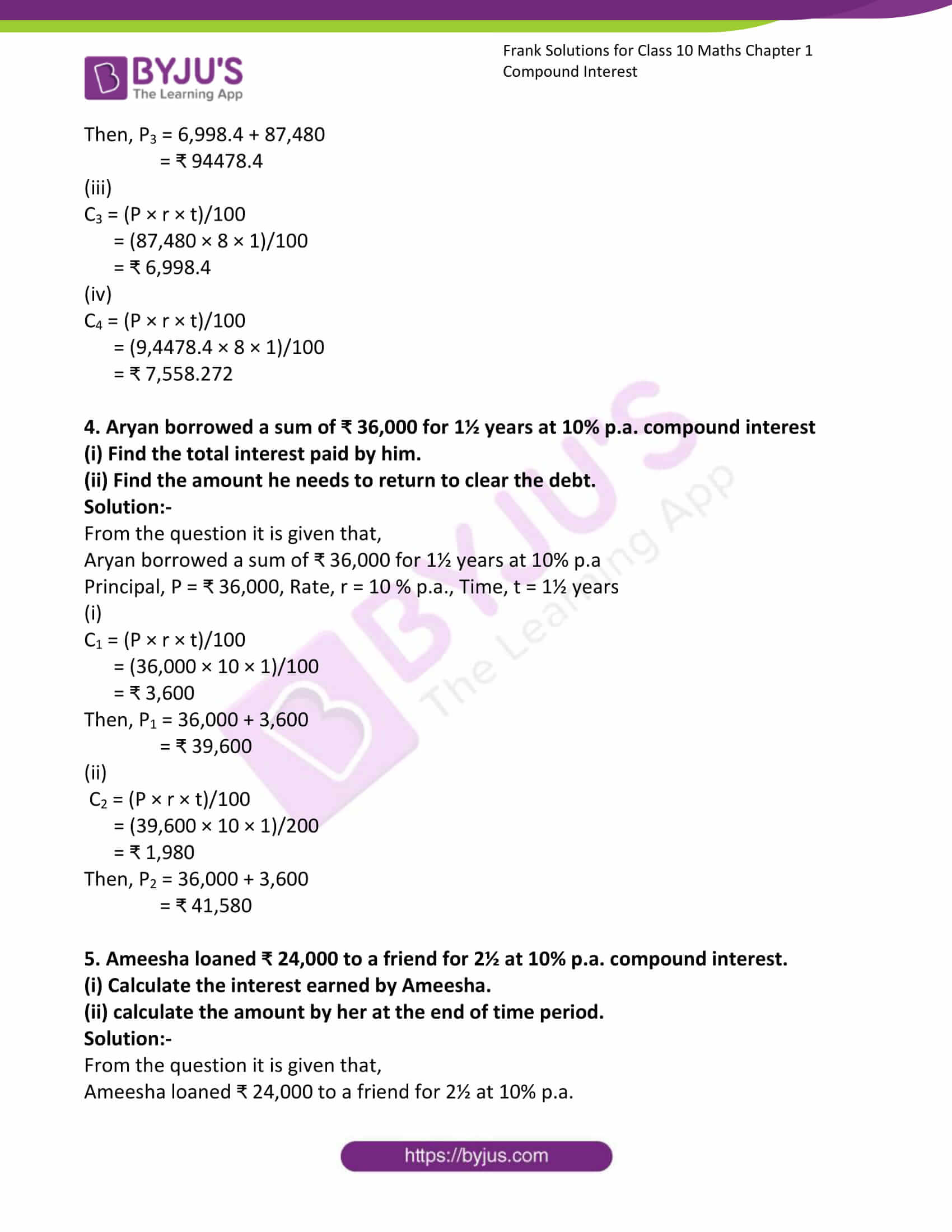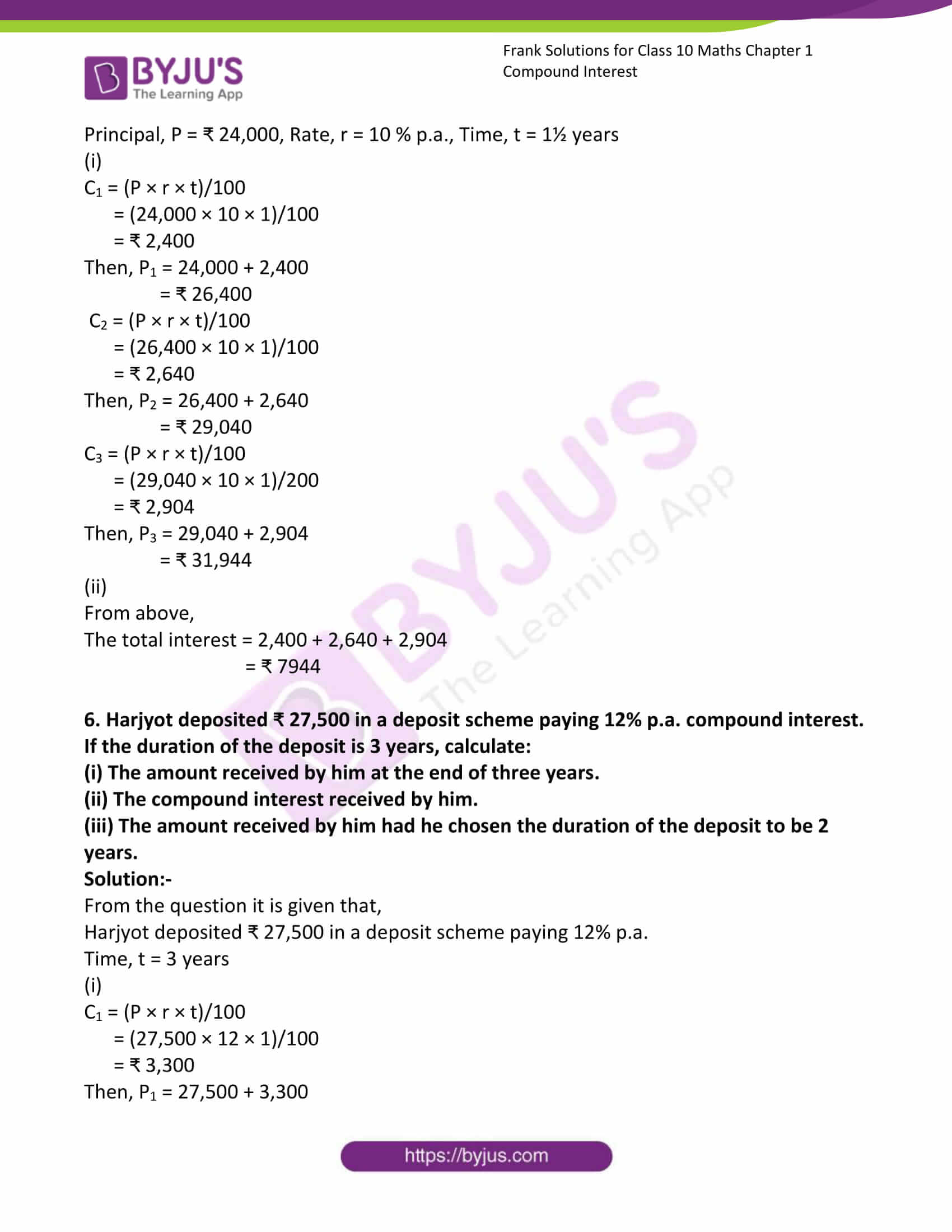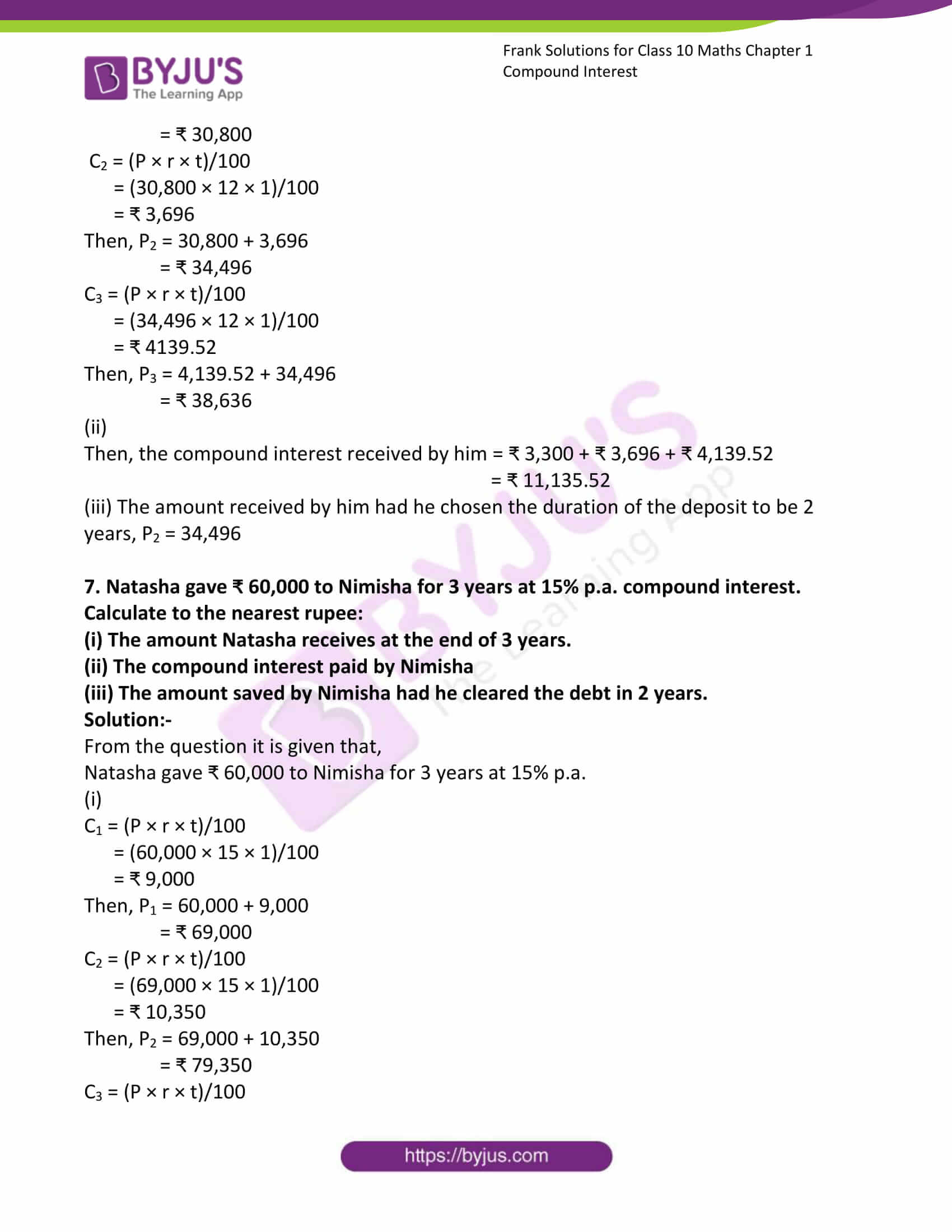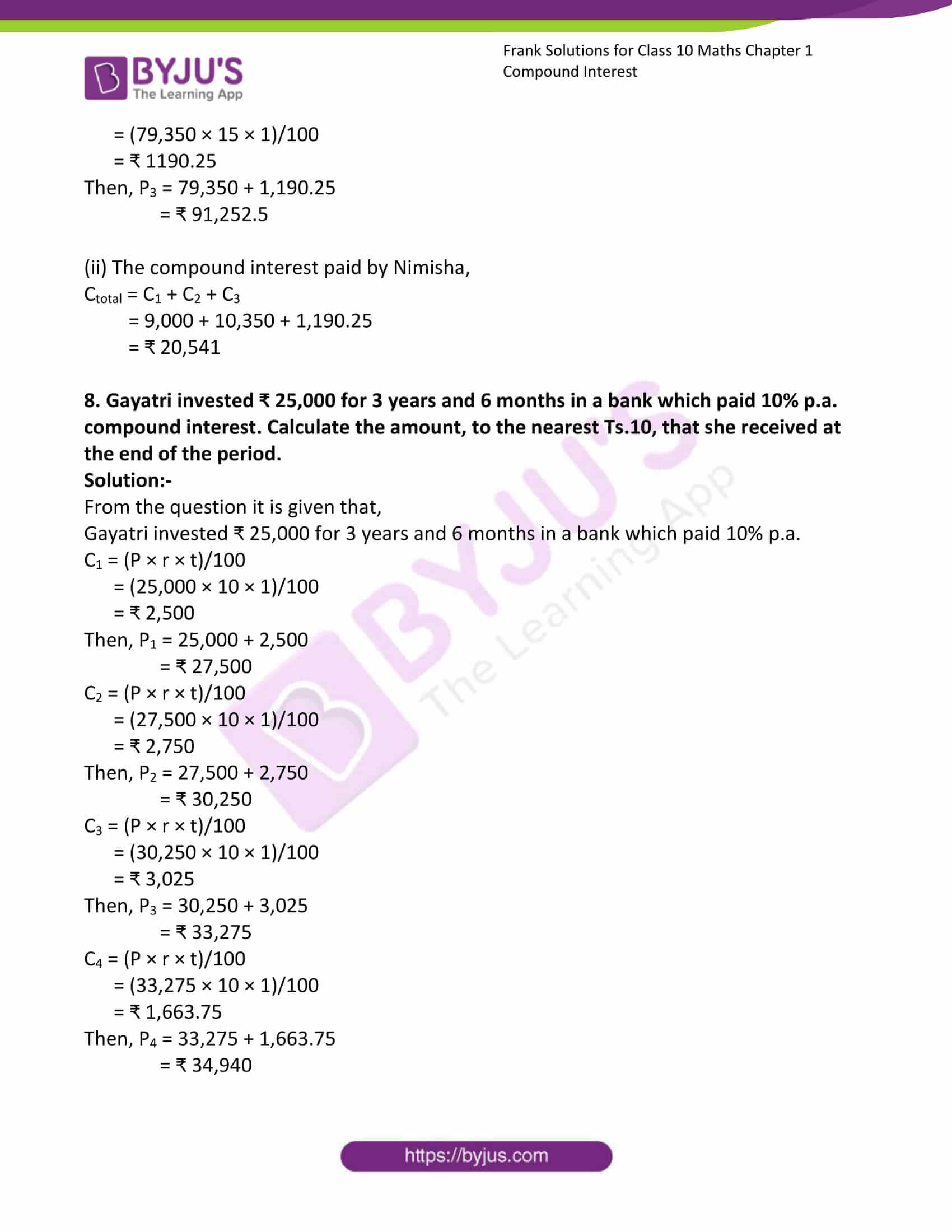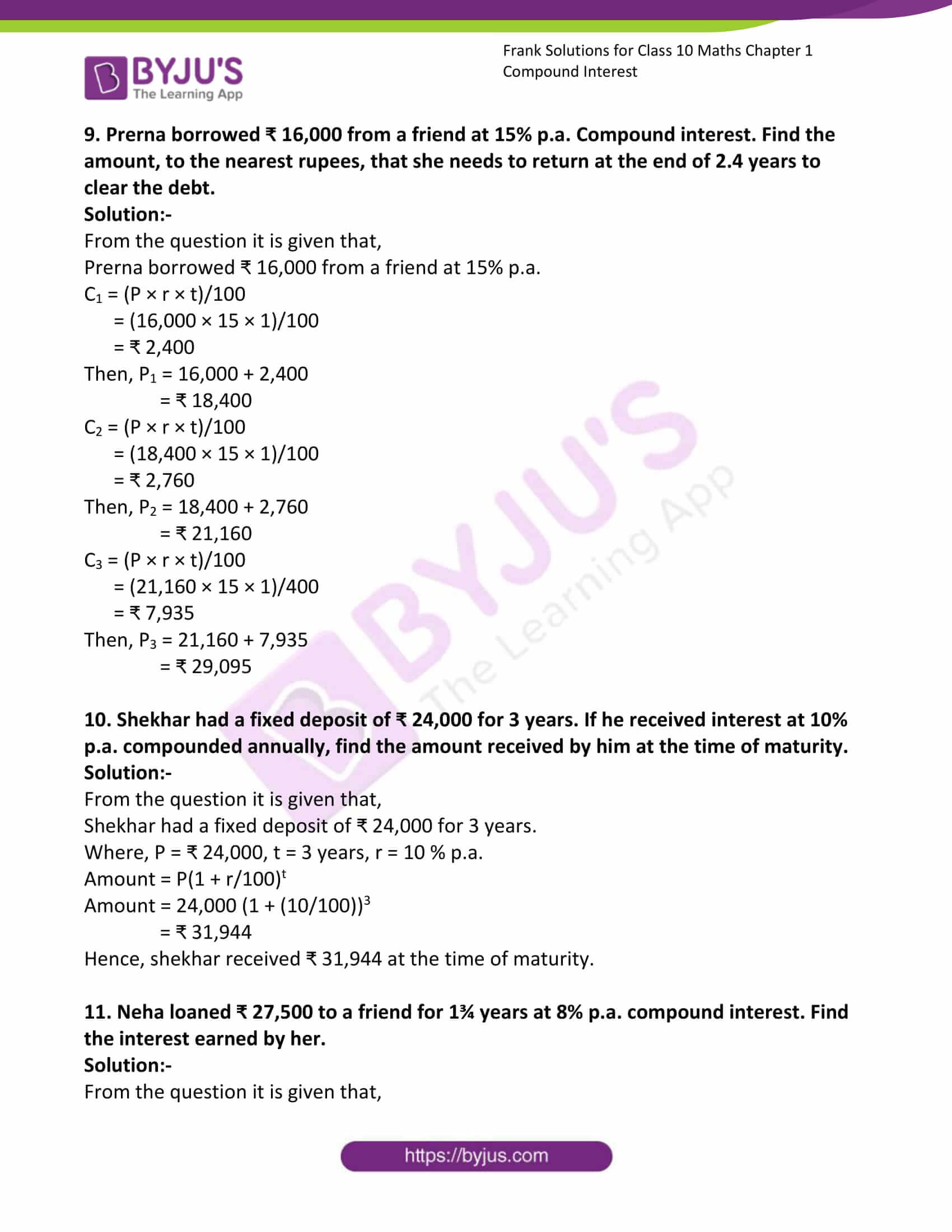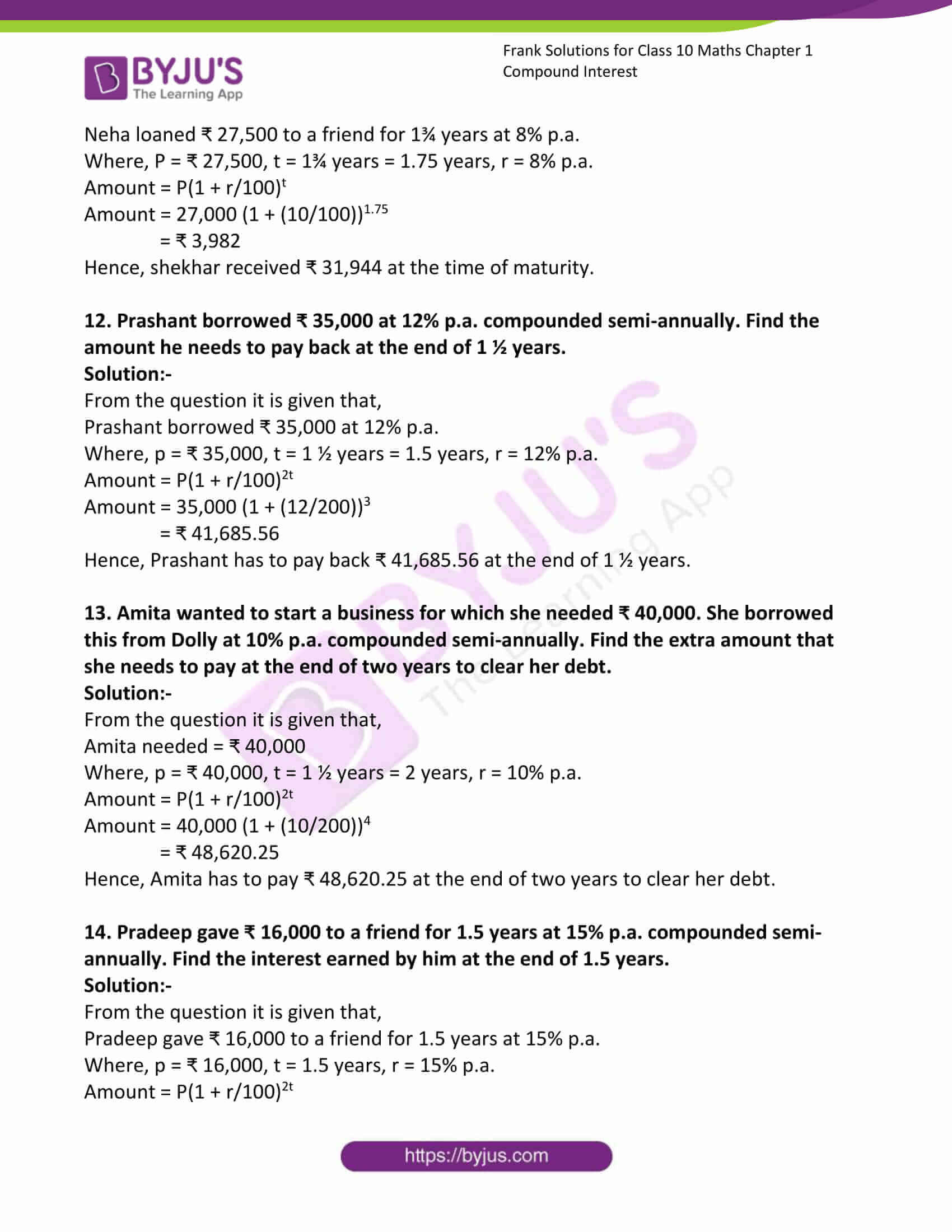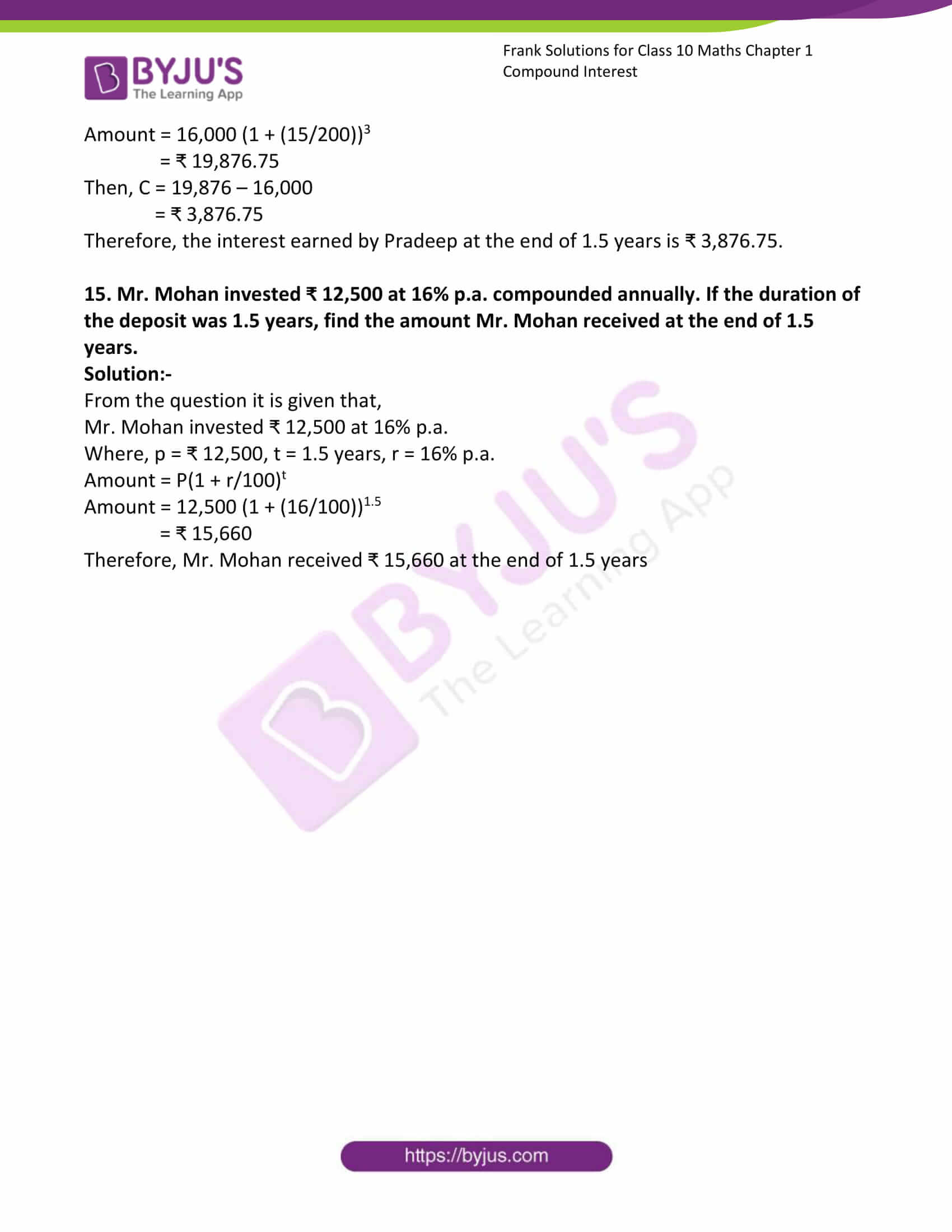### Access Answers to Frank Solutions for Class 10 Maths Chapter 1 Compound Interest

1. Calculate the amount and the compound interest for each of the following:

(a) ₹ 7,500 at 12% p.a. in 3 years.

Solution:-

From the question it is given that,

Principal, P = ₹ 7,500, Rate, r = 12% p.a., Time, t = 3 years

For the first year, t = 1 year

We know that, S.I. = (P × r × t)/100

= (7,500 × 12 × 1)/100

= ₹ 900

Then, A = P + S.I.

= 7,500 + 900

= ₹ 8,400

Therefore, new principal is ₹ 8,400.

Now, for the second year, t = 1 year, p = ₹ 8,400

S.I. = (P × r × t)/100

= (8,400 × 12 × 1)/100

= ₹ 1,008

Then, A = P + S.I.

= 8,400 + 1,008

= ₹ 9,408

Therefore, new principal is ₹ 9,408.

Now, for the second year, t = 1 year, p = ₹ 9,408

S.I. = (P × r × t)/100

= (9,408 × 12 × 1)/100

= ₹ 1,128.96

Then, A = P + S.I.

= 9,408 + 1,128.96

= ₹ 10,536.96

We know that,

C.I. = Interest in first year + interest in second year + interest in third year

= ₹ (900 + 1,008 + 1,128.96)

= ₹ 3,036.96

(b) ₹ 13,500 at 10% p.a. in 2 years.

Solution:-

From the question it is given that,

Principal, P = ₹ 13,500, Rate, r = 10% p.a., Time, t = 2 years

For the first year, t = 1 year

We know that, S.I. = (P × r × t)/100

= (13,500 × 10 × 1)/100

= ₹ 1,350

Then, A = P + S.I.

= 13,500 + 1,350

= ₹ 14,850

Therefore, new principal is ₹ 14,850.

Now, for the second year, t = 1 year, p = ₹ 14,850

S.I. = (P × r × t)/100

= (14,850 × 10 × 1)/100

= ₹ 1,485

Then, A = P + S.I.

= 14,850 + 1,485

= ₹ 16,335

We know that,

C.I. = Interest in first year + interest in second year

= ₹ (1,350 + 1,485)

= ₹ 2,835

(c) ₹ 17,500 at 12% p.a. in 3 years.

Solution:-

From the question it is given that,

Principal, P = ₹ 17,500, Rate, r = 12% p.a., Time, t = 3 years

For the first year, t = 1 year

We know that, S.I. = (P × r × t)/100

= (17,500 × 12 × 1)/100

= ₹ 2,100

Then, A = P + S.I.

= 17,500 + 2,100

= ₹ 19,600

Therefore, new principal is ₹ 19,600.

Now, for the second year, t = 1 year, p = ₹ 19,600

S.I. = (P × r × t)/100

= (8,400 × 12 × 1)/100

= ₹ 1,008

Then, A = P + S.I.

= 19,600 + 2,352

= ₹ 21,952

Therefore, new principal is ₹ 21,952.

Now, for the second year, t = 1 year, p = ₹ 21,952

S.I. = (P × r × t)/100

= (21,952 × 12 × 1)/100

= ₹ 2,634.24

Then, A = P + S.I.

= 21,952 + 2,634.24

= ₹ 7,086.24

We know that,

C.I. = Interest in first year + interest in second year + interest in third year

= ₹ (2,100 + 2,352 + 2,634.24)

= ₹ 7,086.24

(d) ₹ 23,750 at 12% p.a. in 2½ years.

Solution:-

From the question it is given that,

Principal, P = ₹ 23,750, Rate, r = 12% p.a., Time, t = 2½ years

For the first year, t = 1 year

We know that, S.I. = (P × r × t)/100

= (23,750 × 12 × 1)/100

= ₹ 2,850

Then, A = P + S.I.

= 23,750 + 2,850

= ₹ 26,600

Therefore, new principal is ₹ 26,600.

Now, for the second year, t = 1 year, p = ₹ 26,600

S.I. = (P × r × t)/100

= (26,600 × 12 × 1)/100

= ₹ 3,192

Then, A = P + S.I.

= 26,600 + 3,192

= ₹ 29,792

Therefore, new principal is ₹ 29,792.

Now, for the third year, t = ½ year, p = ₹ 29,792.

S.I. = (P × r × t)/100

= (29,792 × 12 × 1)/(100 × 2)

= ₹ 1,787.52

Then, A = P + S.I.

= 29,792 + 1,787.52

= ₹ 31,579.52

We know that,

C.I. = Interest in first year + interest in second year + interest in third year

= ₹ (2,850 + 3,192 + 1,787.52)

= ₹ 7,829.52

(e) ₹ 30,000 at 8% p.a. in 2½ years.

Solution:-

From the question it is given that,

Principal, P = ₹ 30,000, Rate, r = 8% p.a., Time, t = 2½ years

For the first year, t = 1 year

We know that, S.I. = (P × r × t)/100

= (30,000 × 8 × 1)/100

= ₹ 2,400

Then, A = P + S.I.

= 30,000 + 2,400

= ₹ 32,400

Therefore, new principal is ₹ 32,400.

Now, for the second year, t = 1 year, p = ₹ 32,400

S.I. = (P × r × t)/100

= (32,400 × 8 × 1)/100

= ₹ 2,592

Then, A = P + S.I.

= 32,400 + 2,592

= ₹ 34,992

Therefore, new principal is ₹ 34,992.

Now, for the third year, t = ½ year, p = ₹ 34,992.

S.I. = (P × r × t)/100

= (34,992 × 8 × 1)/(100 × 2)

= ₹ 1,399.68

Then, A = P + S.I.

= 34,992 + 1,399.68

= ₹ 36,391.68

We know that,

C.I. = Interest in first year + interest in second year + interest in third year

= ₹ (2,400 + 2,592 + 1,399.68)

= ₹ 6,391.68

(f) ₹ 10,000 at 8% p.a. in 2¼ years.

Solution:-

From the question it is given that,

Principal, P = ₹ 10,000, Rate, r = 8% p.a., Time, t = 2¼ years

For the first year, t = 1 year

We know that, S.I. = (P × r × t)/100

= (10,000 × 8 × 1)/100

= ₹ 800

Then, A = P + S.I.

= 10,000 + 800

= ₹ 10,800

Therefore, new principal is ₹ 10,800.

Now, for the second year, t = 1 year, p = ₹ 10,800

S.I. = (P × r × t)/100

= (10,800 × 8 × 1)/100

= ₹ 864

Then, A = P + S.I.

= 10,800 + 864

= ₹ 11,664

Therefore, new principal is ₹ 11,664.

Now, for the third year, t = ½ year, p = ₹ 11,664.

S.I. = (P × r × t)/100

= (11,664 × 8 × 1)/(100 × 4)

= ₹ 233.28

Then, A = P + S.I.

= 11,664 + 233.28

= ₹ 11,897.28

We know that,

C.I. = Interest in first year + interest in second year + interest in third year

= ₹ (800 + 864 + 233.28)

= ₹ 1,897.28

(g) ₹ 20,000 at 9% p.a. in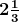years.

Solution:-

From the question it is given that,

Principal, P = ₹ 10,000, Rate, r = 8% p.a., Time, t =years

For the first year, t = 1 year

We know that, S.I. = (P × r × t)/100

= (20,000 × 9 × 1)/100

= ₹ 1,800

Then, A = P + S.I.

= 20,000 + 1,800

= ₹ 21,800

Therefore, new principal is ₹ 21,800.

Now, for the second year, t = 1 year, p = ₹ 21,800

S.I. = (P × r × t)/100

= (21,800 × 9 × 1)/100

= ₹ 1,962

Then, A = P + S.I.

= 21,800 + 1,962

= ₹ 23,762

Therefore, new principal is ₹ 23,762.

Now, for the third year, t = 1/3 year, p = ₹ 23,762.

S.I. = (P × r × t)/100

= (23,762 × 9 × 1)/(100 × 3)

= ₹ 712.86

Then, A = P + S.I.

= 23,762 + 712.86

= ₹ 24,474.86

We know that,

C.I. = Interest in first year + interest in second year + interest in third year

= ₹ (1,800 + 1,962 + 712.86)

= ₹ 4,474.86

(h) ₹ 25,000 at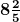% p.a. in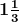years.

Solution:-

From the question it is given that,

Principal, P = ₹ 25,000, Rate, r =% p.a. = 42/5, Time, t =years

For the first year, t = 1 year

We know that, S.I. = (P × r × t)/100

= (25,000 × 42 × 1)/(100 × 5)

= ₹ 2,100

Then, A = P + S.I.

= 25,000 + 2,100

= ₹ 27,100

Therefore, new principal is ₹ 27,100.

Now, for the second year, t = 1/3 year, p = ₹ 27,100

S.I. = (P × r × t)/100

= (27,100 × 42 × 1)/(100 × 5 × 3)

= ₹ 758.80

Then, A = P + S.I.

= 27,100 + 758.80

= ₹ 27,858.80

We know that,

C.I. = Interest in first year + interest in second year

= ₹ (2,100 + 758.80)

= ₹ 2,858.80

(i) ₹ 40,000 at 5¼ p.a. inyears.

Solution:-

From the question it is given that,

Principal, P = ₹ 25,000, Rate, r = 5¼ p.a. = 21/4 %, Time, t =years

For the first year, t = 1 year

We know that, S.I. = (P × r × t)/100

= (40,000 × 21 × 1)/(100 × 4)

= ₹ 2,100

Then, A = P + S.I.

= 40,000 + 2,100

= ₹ 42,100

Therefore, new principal is ₹ 42,100.

Now, for the second year, t = 1/3 year, p = ₹ 42,100

S.I. = (P × r × t)/100

= (42,100 × 21 × 1)/(100 × 4 × 3)

= ₹ 736.75

Then, A = P + S.I.

= 42,100 + 736.75

= ₹ 42,836.75

We know that,

C.I. = Interest in first year + interest in second year

= ₹ (2,100 + 736.75)

= ₹ 2,836.75

(j) ₹ 76,000 at 10% p.a. in 2½ years.

Solution:-

From the question it is given that,

Principal, P = ₹ 10,000, Rate, r = 8% p.a., Time, t = 2½ years

For the first year, t = 1 year

We know that, S.I. = (P × r × t)/100

= (76,000 × 10 × 1)/100

= ₹ 7,600

Then, A = P + S.I.

= 76,000 + 7,600

= ₹ 83,600

Therefore, new principal is ₹ 83,600.

Now, for the second year, t = 1 year, p = ₹ 83,600

S.I. = (P × r × t)/100

= (83,600 × 10 × 1)/100

= ₹ 8,360

Then, A = P + S.I.

= 83,600 + 8,360

= ₹ 91,960

Therefore, new principal is ₹ 91,960.

Now, for the third year, t = ½ year, p = ₹ 91,960.

S.I. = (P × r × t)/100

= (91,960 × 10 × 1)/(100 × 2)

= ₹ 4,598

Then, A = P + S.I.

= 91,960 + 4,598

= ₹ 96,558

We know that,

C.I. = Interest in first year + interest in second year + interest in third year

= ₹ (7,600 + 8,360 + 4,598)

= ₹ 96,558

(k) ₹ 22,500 at 12% p.a. in 1¾ years.

Solution:-

From the question it is given that,

Principal, P = ₹ 22,500, Rate, r = 12% p.a., Time, t = 1¾ years

For the first year, t = 1 year

We know that, S.I. = (P × r × t)/100

= (22,500 × 12 × 1)/100

= ₹ 2,700

Then, A = P + S.I.

= 22,500 + 2,700

= ₹ 25,200

Therefore, new principal is ₹ 25,200.

Now, for the second year, t = ¾ year, p = ₹ 25,200

S.I. = (P × r × t)/100

= (25,200 × 12 × 3)/(100 × 4)

= ₹ 2,268

Then, A = P + S.I.

= 25,200 + 2,268

= ₹ 27,468

We know that,

C.I. = Interest in first year + interest in second year

= ₹ (2,700 + 2,268)

= ₹ 4,968

(i) ₹ 16,000 at 15% p.a. in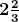years.

Solution:-

From the question it is given that,

Principal, P = ₹ 16,000, Rate, r = 15% p.a., Time, t =years

For the first year, t = 1 year

We know that, S.I. = (P × r × t)/100

= (16,000 × 15 × 1)/100

= ₹ 2,400

Then, A = P + S.I.

= 16,000 + 2,400

= ₹ 18,400

Therefore, new principal is ₹ 18,400.

Now, for the second year, t = 1 year, p = ₹ 18,400

S.I. = (P × r × t)/100

= (18,400 × 15 × 1)/100

= ₹ 2,760

Then, A = P + S.I.

= 18,400 + 2,760

= ₹ 21,160

Therefore, new principal is ₹ 21,160.

Now, for the third year, t = 1/3 year, p = ₹ 21,160.

S.I. = (P × r × t)/100

= (21,160 × 15 × 2)/(100 × 3)

= ₹ 2116

Then, A = P + S.I.

= 21,160 + 2116

= ₹ 23,276

We know that,

C.I. = Interest in first year + interest in second year + interest in third year

= ₹ (2,400 + 2760 + 2116)

= ₹ 7,276

2. A sum of ₹ 65,000 is invested for 3 years at 8% p.a. compound interest.

(i) Find the sum due at the end of the first year.

(ii) Find the sum due at the end of the second year.

(iii) Find the compound interest earned in the first two years.

(iv) Find the compound interest earned in the last year.

Solution:-

From the question it is given that,

Principal, P = ₹ 65,000, Rate, r = 8% p.a., Time, t = 3 years

(i)

C1 = (P × r × t)/100

= (65,000 × 8 × 1)/100

= ₹ 5,200

Then, P1 = 5200 + 65000

= ₹ 70,200

(ii)

C2 = (P × r × t)/100

= (70,200 × 8 × 1)/100

= ₹ 5,616

Then, P2 = 70,200 + 5,616

= ₹ 75,816

(iii)

C1 + C2 = 5,200 + 5,616

= ₹ 10,816

(iv)

C3 = (P × r × t)/100

= (75,816 × 8 × 1)/100

= ₹ 6,065.28

3. Alisha invested ₹ 75,000 for 4 years at 8% p.a. compound interest,

(i) Find the amount at the end of the second year.

(ii) Find the amount at the end of third year.

(iii) Find the interest earned in the third year.

(iv) Calculate the interest for the fourth year.

Solution:-

From the question it is given that,

Alisha invested ₹ 75,000 for 4 years at 8% p.a.

Principal, P = ₹ 75,000, Rate, r = 8% p.a., Time, t = 4 years

(i)

C1 = (P × r × t)/100

= (75,000 × 8 × 1)/100

= ₹ 6,000

Then, P1 = 75,000 + 6,000

= ₹ 81,000

C2 = (P × r × t)/100

= (81,000 × 8 × 1)/100

= ₹ 6,480

Then, P2 = 81,000 + 6,480

= ₹ 87,480

(ii)

C3 = (P × r × t)/100

= (87,480 × 8 × 1)/100

= ₹ 6,998.4

Then, P3 = 6,998.4 + 87,480

= ₹ 94478.4

(iii)

C3 = (P × r × t)/100

= (87,480 × 8 × 1)/100

= ₹ 6,998.4

(iv)

C4 = (P × r × t)/100

= (9,4478.4 × 8 × 1)/100

= ₹ 7,558.272

4. Aryan borrowed a sum of ₹ 36,000 for 1½ years at 10% p.a. compound interest

(i) Find the total interest paid by him.

(ii) Find the amount he needs to return to clear the debt.

Solution:-

From the question it is given that,

Aryan borrowed a sum of ₹ 36,000 for 1½ years at 10% p.a

Principal, P = ₹ 36,000, Rate, r = 10 % p.a., Time, t = 1½ years

(i)

C1 = (P × r × t)/100

= (36,000 × 10 × 1)/100

= ₹ 3,600

Then, P1 = 36,000 + 3,600

= ₹ 39,600

(ii)

C2 = (P × r × t)/100

= (39,600 × 10 × 1)/200

= ₹ 1,980

Then, P2 = 36,000 + 3,600

= ₹ 41,580

5. Ameesha loaned ₹ 24,000 to a friend for 2½ at 10% p.a. compound interest.

(i) Calculate the interest earned by Ameesha.

(ii) calculate the amount by her at the end of time period.

Solution:-

From the question it is given that,

Ameesha loaned ₹ 24,000 to a friend for 2½ at 10% p.a.

Principal, P = ₹ 24,000, Rate, r = 10 % p.a., Time, t = 1½ years

(i)

C1 = (P × r × t)/100

= (24,000 × 10 × 1)/100

= ₹ 2,400

Then, P1 = 24,000 + 2,400

= ₹ 26,400

C2 = (P × r × t)/100

= (26,400 × 10 × 1)/100

= ₹ 2,640

Then, P2 = 26,400 + 2,640

= ₹ 29,040

C3 = (P × r × t)/100

= (29,040 × 10 × 1)/200

= ₹ 2,904

Then, P3 = 29,040 + 2,904

= ₹ 31,944

(ii)

From above,

The total interest = 2,400 + 2,640 + 2,904

= ₹ 7944

6. Harjyot deposited ₹ 27,500 in a deposit scheme paying 12% p.a. compound interest. If the duration of the deposit is 3 years, calculate:

(i) The amount received by him at the end of three years.

(ii) The compound interest received by him.

(iii) The amount received by him had he chosen the duration of the deposit to be 2 years.

Solution:-

From the question it is given that,

Harjyot deposited ₹ 27,500 in a deposit scheme paying 12% p.a.

Time, t = 3 years

(i)

C1 = (P × r × t)/100

= (27,500 × 12 × 1)/100

= ₹ 3,300

Then, P1 = 27,500 + 3,300

= ₹ 30,800

C2 = (P × r × t)/100

= (30,800 × 12 × 1)/100

= ₹ 3,696

Then, P2 = 30,800 + 3,696

= ₹ 34,496

C3 = (P × r × t)/100

= (34,496 × 12 × 1)/100

= ₹ 4139.52

Then, P3 = 4,139.52 + 34,496

= ₹ 38,636

(ii)

Then, the compound interest received by him = ₹ 3,300 + ₹ 3,696 + ₹ 4,139.52

= ₹ 11,135.52

(iii) The amount received by him had he chosen the duration of the deposit to be 2 years, P2 = 34,496

7. Natasha gave ₹ 60,000 to Nimisha for 3 years at 15% p.a. compound interest. Calculate to the nearest rupee:

(i) The amount Natasha receives at the end of 3 years.

(ii) The compound interest paid by Nimisha

(iii) The amount saved by Nimisha had he cleared the debt in 2 years.

Solution:-

From the question it is given that,

Natasha gave ₹ 60,000 to Nimisha for 3 years at 15% p.a.

(i)

C1 = (P × r × t)/100

= (60,000 × 15 × 1)/100

= ₹ 9,000

Then, P1 = 60,000 + 9,000

= ₹ 69,000

C2 = (P × r × t)/100

= (69,000 × 15 × 1)/100

= ₹ 10,350

Then, P2 = 69,000 + 10,350

= ₹ 79,350

C3 = (P × r × t)/100

= (79,350 × 15 × 1)/100

= ₹ 1190.25

Then, P3 = 79,350 + 1,190.25

= ₹ 91,252.5

(ii) The compound interest paid by Nimisha,

Ctotal = C1 + C2 + C3

= 9,000 + 10,350 + 1,190.25

= ₹ 20,541

8. Gayatri invested ₹ 25,000 for 3 years and 6 months in a bank which paid 10% p.a. compound interest. Calculate the amount, to the nearest Ts.10, that she received at the end of the period.

Solution:-

From the question it is given that,

Gayatri invested ₹ 25,000 for 3 years and 6 months in a bank which paid 10% p.a.

C1 = (P × r × t)/100

= (25,000 × 10 × 1)/100

= ₹ 2,500

Then, P1 = 25,000 + 2,500

= ₹ 27,500

C2 = (P × r × t)/100

= (27,500 × 10 × 1)/100

= ₹ 2,750

Then, P2 = 27,500 + 2,750

= ₹ 30,250

C3 = (P × r × t)/100

= (30,250 × 10 × 1)/100

= ₹ 3,025

Then, P3 = 30,250 + 3,025

= ₹ 33,275

C4 = (P × r × t)/100

= (33,275 × 10 × 1)/100

= ₹ 1,663.75

Then, P4 = 33,275 + 1,663.75

= ₹ 34,940

9. Prerna borrowed ₹ 16,000 from a friend at 15% p.a. Compound interest. Find the amount, to the nearest rupees, that she needs to return at the end of 2.4 years to clear the debt.

Solution:-

From the question it is given that,

Prerna borrowed ₹ 16,000 from a friend at 15% p.a.

C1 = (P × r × t)/100

= (16,000 × 15 × 1)/100

= ₹ 2,400

Then, P1 = 16,000 + 2,400

= ₹ 18,400

C2 = (P × r × t)/100

= (18,400 × 15 × 1)/100

= ₹ 2,760

Then, P2 = 18,400 + 2,760

= ₹ 21,160

C3 = (P × r × t)/100

= (21,160 × 15 × 1)/400

= ₹ 7,935

Then, P3 = 21,160 + 7,935

= ₹ 29,095

10. Shekhar had a fixed deposit of ₹ 24,000 for 3 years. If he received interest at 10% p.a. compounded annually, find the amount received by him at the time of maturity.

Solution:-

From the question it is given that,

Shekhar had a fixed deposit of ₹ 24,000 for 3 years.

Where, P = ₹ 24,000, t = 3 years, r = 10 % p.a.

Amount = P(1 + r/100)t

Amount = 24,000 (1 + (10/100))3

= ₹ 31,944

Hence, shekhar received ₹ 31,944 at the time of maturity.

11. Neha loaned ₹ 27,500 to a friend for 1¾ years at 8% p.a. compound interest. Find the interest earned by her.

Solution:-

From the question it is given that,

Neha loaned ₹ 27,500 to a friend for 1¾ years at 8% p.a.

Where, P = ₹ 27,500, t = 1¾ years = 1.75 years, r = 8% p.a.

Amount = P(1 + r/100)t

Amount = 27,000 (1 + (10/100))1.75

= ₹ 3,982

Hence, shekhar received ₹ 31,944 at the time of maturity.

12. Prashant borrowed ₹ 35,000 at 12% p.a. compounded semi-annually. Find the amount he needs to pay back at the end of 1 ½ years.

Solution:-

From the question it is given that,

Prashant borrowed ₹ 35,000 at 12% p.a.

Where, p = ₹ 35,000, t = 1 ½ years = 1.5 years, r = 12% p.a.

Amount = P(1 + r/100)2t

Amount = 35,000 (1 + (12/200))3

= ₹ 41,685.56

Hence, Prashant has to pay back ₹ 41,685.56 at the end of 1 ½ years.

13. Amita wanted to start a business for which she needed ₹ 40,000. She borrowed this from Dolly at 10% p.a. compounded semi-annually. Find the extra amount that she needs to pay at the end of two years to clear her debt.

Solution:-

From the question it is given that,

Amita needed = ₹ 40,000

Where, p = ₹ 40,000, t = 1 ½ years = 2 years, r = 10% p.a.

Amount = P(1 + r/100)2t

Amount = 40,000 (1 + (10/200))4

= ₹ 48,620.25

Hence, Amita has to pay ₹ 48,620.25 at the end of two years to clear her debt.

14. Pradeep gave ₹ 16,000 to a friend for 1.5 years at 15% p.a. compounded semi-annually. Find the interest earned by him at the end of 1.5 years.

Solution:-

From the question it is given that,

Pradeep gave ₹ 16,000 to a friend for 1.5 years at 15% p.a.

Where, p = ₹ 16,000, t = 1.5 years, r = 15% p.a.

Amount = P(1 + r/100)2t

Amount = 16,000 (1 + (15/200))3

= ₹ 19,876.75

Then, C = 19,876 – 16,000

= ₹ 3,876.75

Therefore, the interest earned by Pradeep at the end of 1.5 years is ₹ 3,876.75.

15. Mr. Mohan invested ₹ 12,500 at 16% p.a. compounded annually. If the duration of the deposit was 1.5 years, find the amount Mr. Mohan received at the end of 1.5 years.

Solution:-

From the question it is given that,

Mr. Mohan invested ₹ 12,500 at 16% p.a.

Where, p = ₹ 12,500, t = 1.5 years, r = 16% p.a.

Amount = P(1 + r/100)t

Amount = 12,500 (1 + (16/100))1.5

= ₹ 15,660

Therefore, Mr. Mohan received ₹ 15,660 at the end of 1.5 years